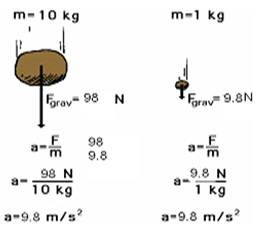### Forces and Laws of Motion - Revision Notes

CBSE Class 9 Science

Revision Notes
CHAPTER – 9
Force & Laws Of Motion

Balanced and Unbalanced Forces

Balanced Forces: The net force is when two or more forces are applied on the same object and at the same time. The applied forces combined are called the net force.

Balanced Forces The force I apply in one direction plus the force you apply in the opposite direction are added together. 2 Because the forces are equal and balanced

Unbalanced Forces: A force is applied in one direction and either another smaller or larger force is applied in the opposite direction or no force is applied at all in the opposite direction.

Unbalanced Forces If I have a chair and I push on one side of it with a force of 50 N and you push on the other side, with a force of 25 N, will the chair move? Which way will it move? The direction in which the most force is applied. What is the net force? 50 N 25 N.

2. Laws of Motion

Newton's First Law

1st Law – An object at rest will stay at rest, and an object in motion will stay in motion at constant velocity, unless acted upon by an unbalanced force.

An object at rest will stay at rest, and an object in motion will stay in motion at constant velocity, unless acted upon by an unbalanced force.

Newton's Second Law

"If the net force on an object is not zero, the object will accelerate. The direction of the acceleration is the same as the direction of the net force. The magnitude of the acceleration is directly proportional to the net force applied, and inversely proportional to the mass of the object."

Mathematical symbols provide a convenient shorthand for all of this:

$a=\frac{{F}_{net}}{m}\phantom{\rule{thickmathspace}{0ex}}\phantom{\rule{thickmathspace}{0ex}}or\phantom{\rule{thickmathspace}{0ex}}\phantom{\rule{thickmathspace}{0ex}}{F}_{net}=ma$The Effect of Mass

A force applied to an automobile will not have the same effect as the same force applied to a pencil. An automobile resists accelerating much more than a pencil does, because it has more inertia, or mass.

The acceleration of an object depends not only on how hard you push on it, but also on how much the object resists being pushed.

What is the effect of mass on acceleration? This, too, turns out to be quite simple (I wonder why...). For the same force, an object with twice the mass will have half the acceleration. If it had three times the mass, the same force will produce one-third the acceleration. Four times the mass gives one-fourth of the acceleration, and so on.

This type of relationship between quantities (double one, get half the other) is called an inverse proportion or inverse variation. In other words, then:

Newton’s Second Law of Motion The acceleration of an object is dependent upon both force and mass. Thus, if the colliding objects have unequal mass, they will have unequal accelerations as a result of the contact force which results during the collision.

Newton's Third Law

Newton's Third Law is stated as: For every action there is an equal and opposite reaction.

"Action...Reaction" means that forces always occur in pairs. (Forces are interactions between objects, like conversations are interactions between people.)

Single, isolated forces never happen. The two forces involved are called the "action force" and the "reaction force."

These names are unfortunate for a couple of reasons :

Either force in an interaction can be the "action" force or the "reaction" force

The action and reaction forces exist at the same time.

"Equal" Means

Both forces are exactly the same size. They are equal in magnitude. Both forces exist at exactly the same time. They both start at exactly the same instant, and they both stop at exactly the same instant. They are equal in time.

"Opposite" means that the two forces always act in opposite directions - exactly ${180}^{0}$ apart.

Newton's third law of motion In every interaction, there is a pair of forces acting on the two interacting objects. The size of the force on the first object equals the size of the force on the second object. The direction of the force on the first object is opposite to the direction of the force on the second object. Forces always come in pairs - equal and opposite action-reaction force pairs.

Newton's third law of motion applied to collisions between two objects. In a collision between two objects, both objects experience forces which are equal in magnitude and opposite in direction. Such forces cause one object to speed up (gain momentum) and the other object to slow down (lose momentum). According to Newton's third law, the forces on the two objects are equal in magnitude.

3. Inertia and Mass

Inertia is the tendency of an object to resist any change in its motion. An object will continue to move at the same speed in the same direction unless acted upon by an unbalanced force. Inertia & Mass Inertia & Mass of a bowling ball rolled down the road would eventually come to a stop. Friction is an unbalanced force that causes the ball to stop or slow down. Without friction, the ball would keep going.

Mass is the amount of matter in an object. A bowling ball has more mass than a tennis ball. The greater the mass of an object the greater its inertia.

Mass is the measurement of inertia.

4. Conservation of Momentum

Law of Conservation of Momentum

In a closed system, the vector sum of the momenta before and after an impact must be equal.

Before After

= ${m}_{1}{v}_{1}^{\prime }$ + ${m}_{2}{v}_{2}^{\prime }$

Internal and External Forces